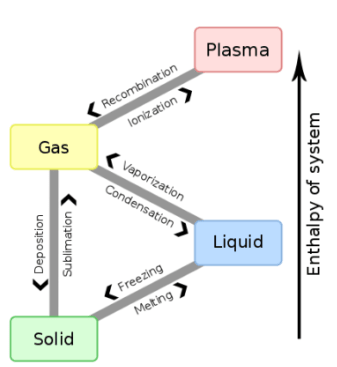Thermodynamics

# Difference between latent heat of fusion and latent heat of vaporization

The latent heat of fusion is the heat required to change the solid from solid to liquid state without any change in temperature. The latent heat of fusion of ice is 336000 J/kg. While Latent heat of vaporization is the heat required to change the liquid from liquid to vapor state without any change in temperature.

## What is latent heat?

The latent heat is one that “feels” as it represents the thermal energy is released or absorbed during a phase change, without increasing or decreasing the temperature of the thermodynamic system. There are several types of latent heat, which are governed by the phase changes of a substance.
The types of latent heat are the latent heat of fusion, vaporization, solidification, and condensation. In other words, these values ​​are the units of heat per mass that are required to achieve the phase change. In the field of thermodynamics, the study of heat transfer and thermal effects is common.Latent heat These effects are involved in any process, even those that occur at a constant temperature. The two types of heat that can be transferred to a body or substance and to the surrounding environment during a process are then observed, which are governed by the individual properties of the substance involved: sensible heat and latent heat.
Sensitive heat is heat that refers to heat that is ” felt”  or measured in the process through changes in body temperature. Instead, latent heat refers to the moment in which energy is absorbed or released without generating temperature changes.

## What is Latent Heat of Fusion?The heat energy required to change a unit mass of a substance from solid to liquid state at its melting point without a change in its temperature is called its latent heat of fusion. It is denoted By ( Hf ).
When a substance is changed from solid to liquid state by adding heat, the process is called melting or fusion. The temperature at which a solid starts melting is called its fusion point or melting point.
When a process is reversed i.e., when a liquid is cooled, it changes into a solid state. The temperature at which a substance changes from liquid to solid state is called its freezing point.
Different substances have different melting points. However, the freezing point of a substance is the same as its melting point.
It is denoted by Hf.
Hf = ΔQf/ m
or   Δ Qf  = m Hf … …. … … (4)

### Example of latent heat of fusion

• Ice changes at 0° C into water. The latent heat of fusion of ice is 3.36 × 105 J kg -1. That is; 3.36 × 105 Joule heat is required to melt 1 kg of ice into the water at 0°C.

## What is Latent heat of vaporization?

The heat energy required to change a unit mass of a substance from liquid to a gaseous state at its boiling point without a change in its temperature is called the latent heat of vaporization. It is represented by H v. Its S.I unit is J/kg. Its formula is given as:
Hv = ΔQv/m

### Example of latent heat of vaporization

When water is heated, it boils at 100 °C under standard pressure. Its temperature remains 100 °C until it is changed completely into steam. Its latent heat of vaporization is 2260000 J/kg. That is one kilogram of water requires 2260000 Joule heat to change it completely into steam at its boiling point.

## Table of latent heat of vaporization of some substances

 Substance Heat of vaporisation (Hv) in kJ/kg Aluminium 10500 Copper 4810 Gold 1580 Helium 21 Lead 858 Mercury 270 Nitrogen 200 Oxygen 210 Water 2260

## Latent heat of solidification

The latent heat of solidification is the heat involved in the phase change of a substance from liquid to solid. As previously stated, the molecules of a substance in the liquid phase have more internal energy than solid ones, so in solidification energy is released instead of absorbing it, as an infusion.
So, in a thermodynamic system, it can be said that the latent heat of solidification is the opposite of that of fusion since the energy involved is released to the outside when the phase change occurs.
In other words, if the value of the latent heat of fusion of water is 333.55 kJ / Kg, then the value of the latent heat of solidification or freezing of water will be -333.55 kJ / Kg.

## Latent heat of condensation

The latent heat of condensation is that which occurs when there is a phase change of a substance from gaseous to liquid, as in the case of water vapor.
Regarding the energy of each molecule, in gases it is even greater than in liquids, so an energy release also occurs when passing from the first phase to the second.
Again, it can be said that the value of the latent heat of condensation will be the same as that of vaporization but with a negative value. So, a latent heat condensation value for water will be -2257 kJ / Kg.
At higher temperatures, the heat of condensation will decrease, while the boiling point will increase.